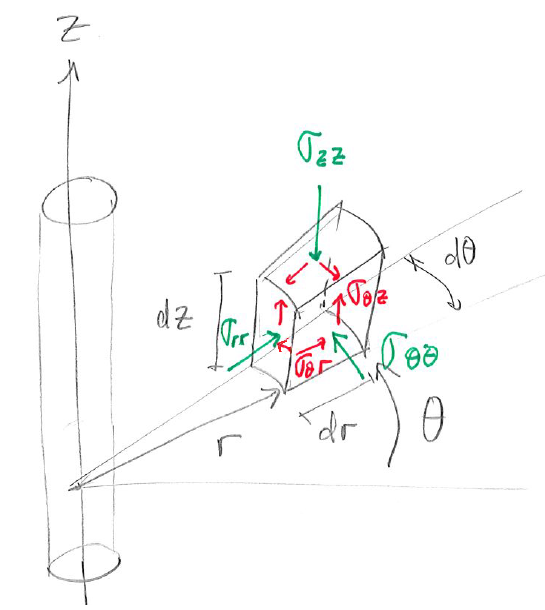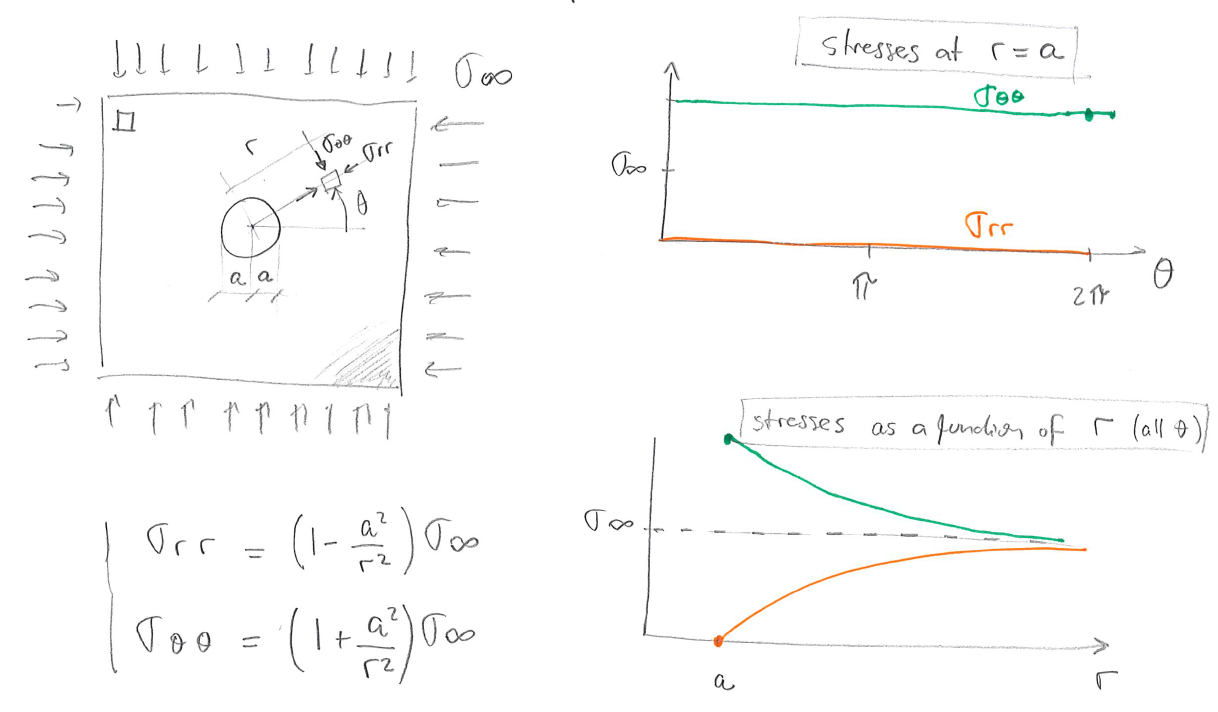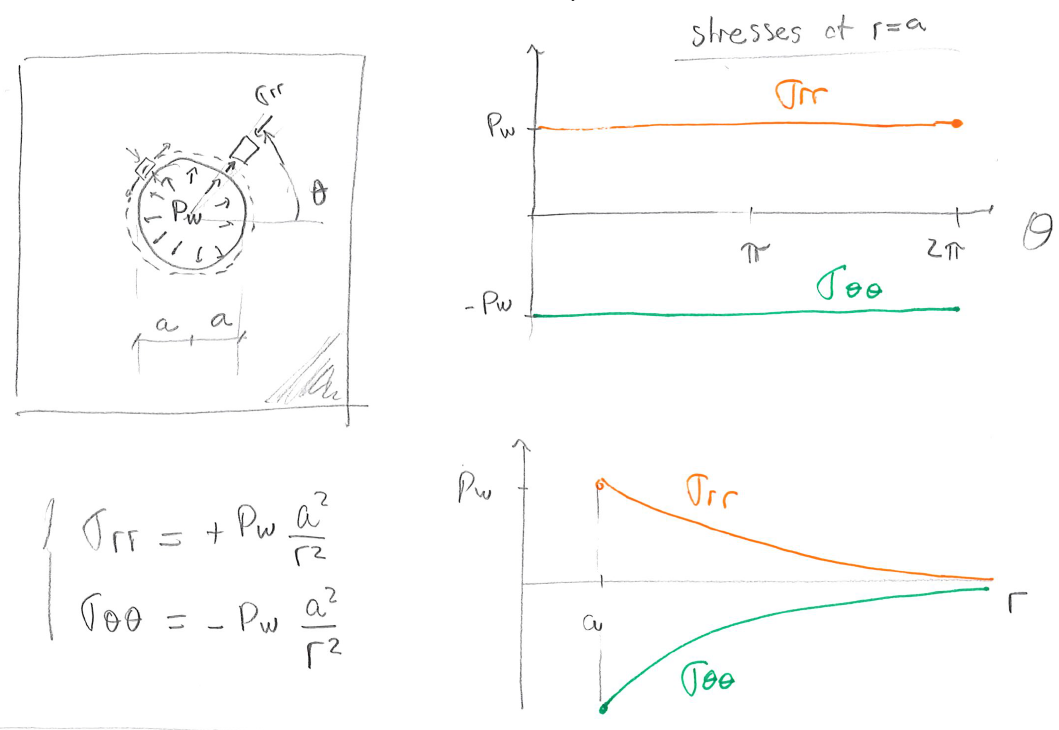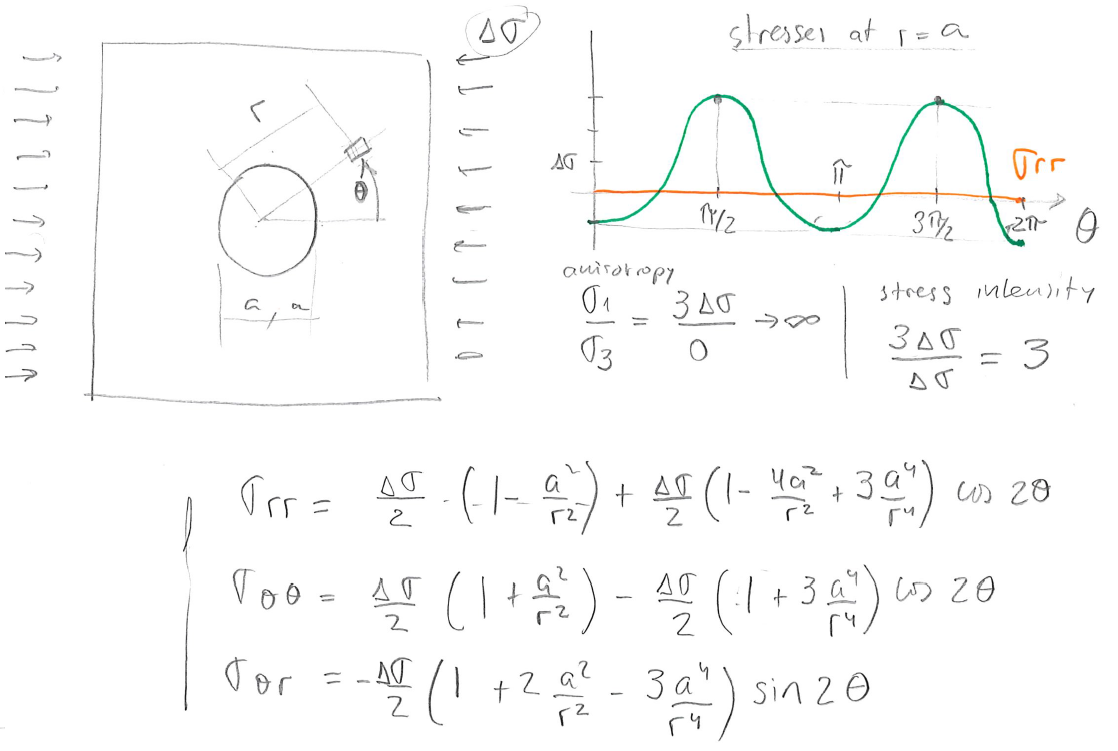Subsections

# 6.2 Kirsch solution for stresses around a cylindrical cavity

## 6.2.1 Cylindrical coordinate system

The cylindrical symmetry of a wellbore prompts the utilization of a cylindrical coordinate system rather than a rectangular cartesian coordinate system. The volume element of stresses in cylindrical coordinates is shown in Fig. 6.4. The distanceis measured from the center axis of the wellbore. The angleis measured with respect to a predefined plane.The normal stresses are radial stress, tangential or hoop stress, and axial stress. The shear stresses are,, and.

## 6.2.2 Kirsch solution components

The Kirsch solution allows us to calculate normal and shear stresses around a circular cavity in a homogeneous linear elastic solid . The complete Kirsch solution assumes independent action of multiple factors, namely far-field isotropic stress, deviatoric stress, wellbore pressure and pore pressure.

1. Isotropic far-field stress: The solution for a compressive isotropic far field stressis shown in Fig. 6.5. The presence of the wellbore amplifies compressive stresses 2 timesall around the wellbore wall in circumferential direction. The presence of the wellbore cavity also creates infinitely large stress anisotropy at the wellbore wallall around the wellbore wall, sincein this case. Stresses decrease inversely proportional toand are neglible at4 radii from the wellbore wall.2. Inner wellbore pressure: The solution for fluid wall pressure in the wellboreis shown in Fig. 6.6. We assume a non-porous solid now. This assumption will be relaxed later on. Wellbore pressure adds compression on the wellbore wall, and induces cavity expansion and tensile hoop stressesall around the wellbore.3. Deviatoric stress: The solution for a deviatoric stressaligned withis shown in Fig. 6.7. The deviatoric stress results in compression on the wellbore wallatand, and in tensionatand. Hence, the presence of the wellbore amplifies compressive stresses 3 timesatand. The variation of stresses around the wellbore depend on harmonic functionsand.4. Pore pressure: The last step consists in assuming a perfect mud-cake, so that, the effective stress wall support (as shown in Fig. 6.6) isinstead of.

## 6.2.3 Complete Kirsch solution

Consider a vertical wellbore subjected to horizontal stressesand, both principal stresses, vertical stress, pore pressure, and wellbore pressure. The corresponding effective in-situ stresses are,, and. The Kirsch solution for a wellbore with radiuswithin a linear elastic and isotropic solid is:(6.2)

whereis the radial effective stress,is the tangential (hoop) effective stress,is the shear stress in a plane perpendicular toin tangential direction, andis the vertical effective stress in direction. The angleis the angle between the direction ofand the point at which stress is considered. The distanceis measured from the center of the wellbore. For example, at the wellbore wall.

An example of the solution of Kirsch equations forMPa,MPa, andMPa is available in Figure 6.8. The plots show radialand tangentialeffective stresses, as well as the calculated principal stressesand.Let us obtainandat the wellbore wall. The radial stress for allis(6.3)

The hoop stress depends on,(6.4)

and it is the minimum atand(azimuth of) and the maximum atand(azimuth of):(6.5)

These locations will be prone to develop tensile fractures (and) and shear fractures (and). The shear stress around the wellbore wall is. This makes sense because fluids (drilling mud) cannot apply steady shear stresses on the surface of a solid. Finally, the effective vertical stress is(6.6)